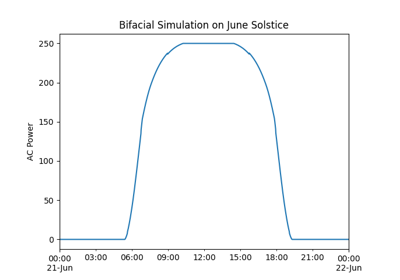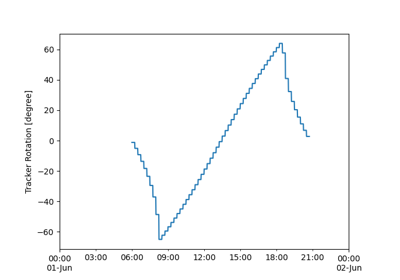# pvlib.pvsystem.SingleAxisTrackerMount#

class pvlib.pvsystem.SingleAxisTrackerMount(axis_tilt: float = 0.0, axis_azimuth: float = 0.0, max_angle: = 90.0, backtrack: bool = True, gcr: float = 0.2857142857142857, cross_axis_tilt: float = 0.0, racking_model: = None, module_height: = None)[source]#

Single-axis tracker racking for dynamic solar tracking.

Parameters
• axis_tilt (float, default 0) – The tilt of the axis of rotation (i.e, the y-axis defined by axis_azimuth) with respect to horizontal. [degrees]

• axis_azimuth (float, default 180) – A value denoting the compass direction along which the axis of rotation lies, measured east of north. [degrees]

• max_angle (float or tuple, default 90) –

A value denoting the maximum rotation angle, in decimal degrees, of the one-axis tracker from its horizontal position (horizontal if axis_tilt = 0). If a float is provided, it represents the maximum rotation angle, and the minimum rotation angle is assumed to be the opposite of the maximum angle. If a tuple of (min_angle, max_angle) is provided, it represents both the minimum and maximum rotation angles.

A rotation to ‘max_angle’ is a counter-clockwise rotation about the y-axis of the tracker coordinate system. For example, for a tracker with ‘axis_azimuth’ oriented to the south, a rotation to ‘max_angle’ is towards the west, and a rotation toward ‘min_angle’ is in the opposite direction, toward the east. Hence a max_angle of 180 degrees (equivalent to max_angle = (-180, 180)) allows the tracker to achieve its full rotation capability.

• backtrack (bool, default True) – Controls whether the tracker has the capability to “backtrack” to avoid row-to-row shading. False denotes no backtrack capability. True denotes backtrack capability.

• gcr (float, default 2.0/7.0) – A value denoting the ground coverage ratio of a tracker system which utilizes backtracking; i.e. the ratio between the PV array surface area to total ground area. A tracker system with modules 2 meters wide, centered on the tracking axis, with 6 meters between the tracking axes has a gcr of 2/6=0.333. If gcr is not provided, a gcr of 2/7 is default. gcr must be <=1. [unitless]

• cross_axis_tilt (float, default 0.0) – The angle, relative to horizontal, of the line formed by the intersection between the slope containing the tracker axes and a plane perpendicular to the tracker axes. Cross-axis tilt should be specified using a right-handed convention. For example, trackers with axis azimuth of 180 degrees (heading south) will have a negative cross-axis tilt if the tracker axes plane slopes down to the east and positive cross-axis tilt if the tracker axes plane slopes up to the east. Use `calc_cross_axis_tilt()` to calculate cross_axis_tilt. [degrees]

• racking_model (str, optional) – Valid strings are ‘open_rack’, ‘close_mount’, and ‘insulated_back’. Used to identify a parameter set for the SAPM cell temperature model.

• module_height (float, optional) – The height above ground of the center of the module [m]. Used for the Fuentes cell temperature model.

Methods

 `__init__`([axis_tilt, axis_azimuth, ...]) `get_orientation`(solar_zenith, solar_azimuth) Determine module orientation.

Attributes

 `axis_azimuth` `axis_tilt` `backtrack` `cross_axis_tilt` `gcr` `max_angle` `module_height` `racking_model`

## Examples using `pvlib.pvsystem.SingleAxisTrackerMount`#Bifacial Modeling - modelchain

Bifacial Modeling - modelchainDiscontinuous Tracking

Discontinuous Tracking